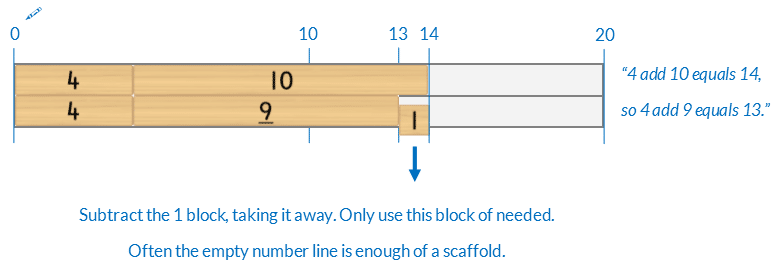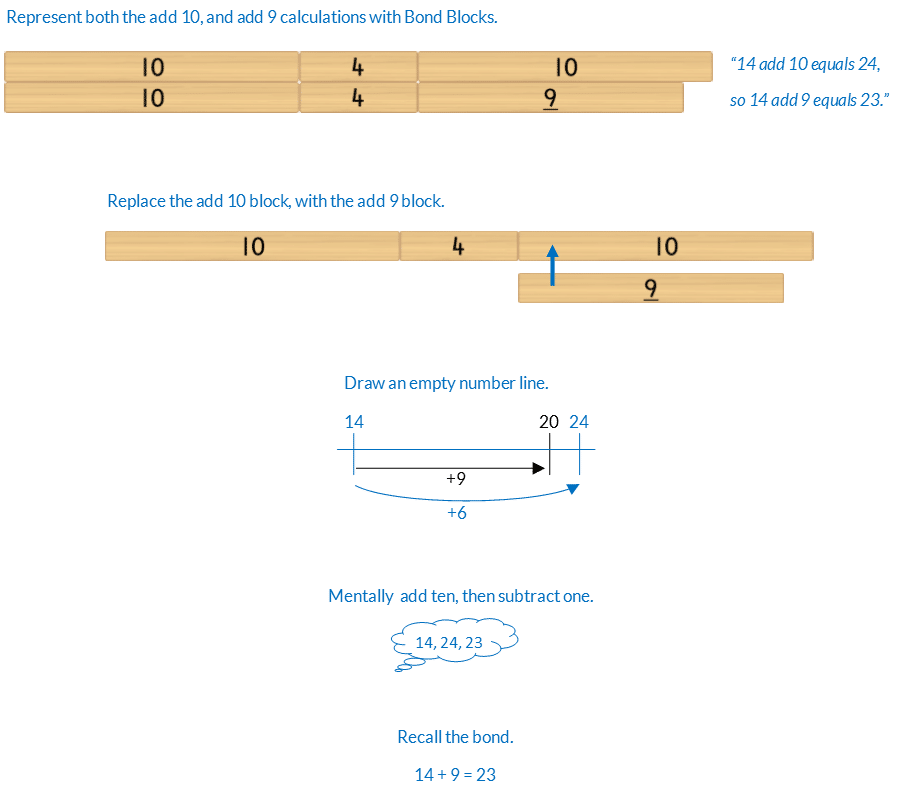## 69) Near Ten

Ten Plus Bonds: Strategy 9+### Mathematics

To develop the strategy of adding 9, by adding 10, then subtracting one, for wholes less than 20.

For example, to solve 4 + 9Prior knowledge of ten plus bonds, the commutative property of addition and adding and subtracting ten and one is needed.

### Language

• equals## Differentiation

### A little easier

##### Represent the subtract one

Make the subtracting one explicit by:

• representing the one with a 1 block and
• change the empty frames into an empty number line.For example, 4 + 9### A little harder

##### Near ten strategy +9

Play Bonds of 11 to 20: Near Ten Strategy +9 a little harder. In the game students have to add nine to parts that are teen numbers using the same strategy.##### Reduce scaffolds

The blocks are a scaffold to support calculation. If students can calculate without some or all of the blocks encourage this. Below is a progression of reducing scaffolding, from most to least support, using near ten for 14 + 9.### Progression

In the next activity students extend the near 10 strategy to subtracting a part of 11. Go to

##### Activity 51

Ten Plus Bonds: Bridging Ten Addition, Strategy 8+